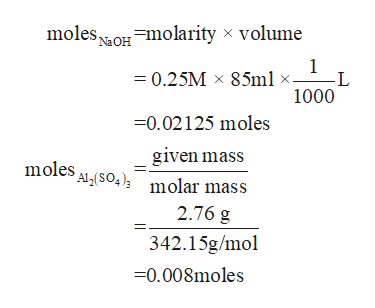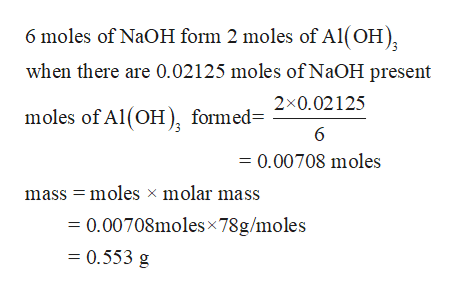# 1. When a solution of aluminum sulfate and sodium hydroxide are mixed, a white gelatinousprecipitate forms.a. What is the mass of the precipitate when 2.76g of aluminum sulfate in 125mL of solution iscombined with 85.0 mL of 0.2500 M sodium hydroxide?b. What is the molarity of cation in excess? Ignore the spectator ions and assume volume isadditive).

Question
6 views

1. When a solution of aluminum sulfate and sodium hydroxide are mixed, a white gelatinous
precipitate forms.
a. What is the mass of the precipitate when 2.76g of aluminum sulfate in 125mL of solution is
combined with 85.0 mL of 0.2500 M sodium hydroxide?
b. What is the molarity of cation in excess? Ignore the spectator ions and assume volume is

check_circle

Step 1

a) The equation for the reaction is

Step 2

Moles of NaOH and Al2(SO4)3help_outlineImage Transcriptionclosemoles =molarityx volume Na OH 1 -L 1000 =0.25M 85ml 0.02125 moles given mass Alg(S04) molar mass moles 2.76 g 342.15g/mol -0.008moles fullscreen
Step 3

Since 1 mole of aluminum sulphate reacts with 6 moles of NaOH, the mole ratio is 1:6.

The mole ratio of the moles obtain...help_outlineImage Transcriptionclose6 moles of NaOH form 2 moles of Al(OH) when there are 0.02125 moles of NaOH present 2x0.02125 moles of Al(OH, formed 6 = 0.00708 moles mass moles x molar mass = 0.00708molesx 78g/moles = 0.553 g fullscreen

### Want to see the full answer?

See Solution

#### Want to see this answer and more?

Solutions are written by subject experts who are available 24/7. Questions are typically answered within 1 hour.*

See Solution
*Response times may vary by subject and question.
Tagged in
ScienceChemistry

### Quantitative analysis